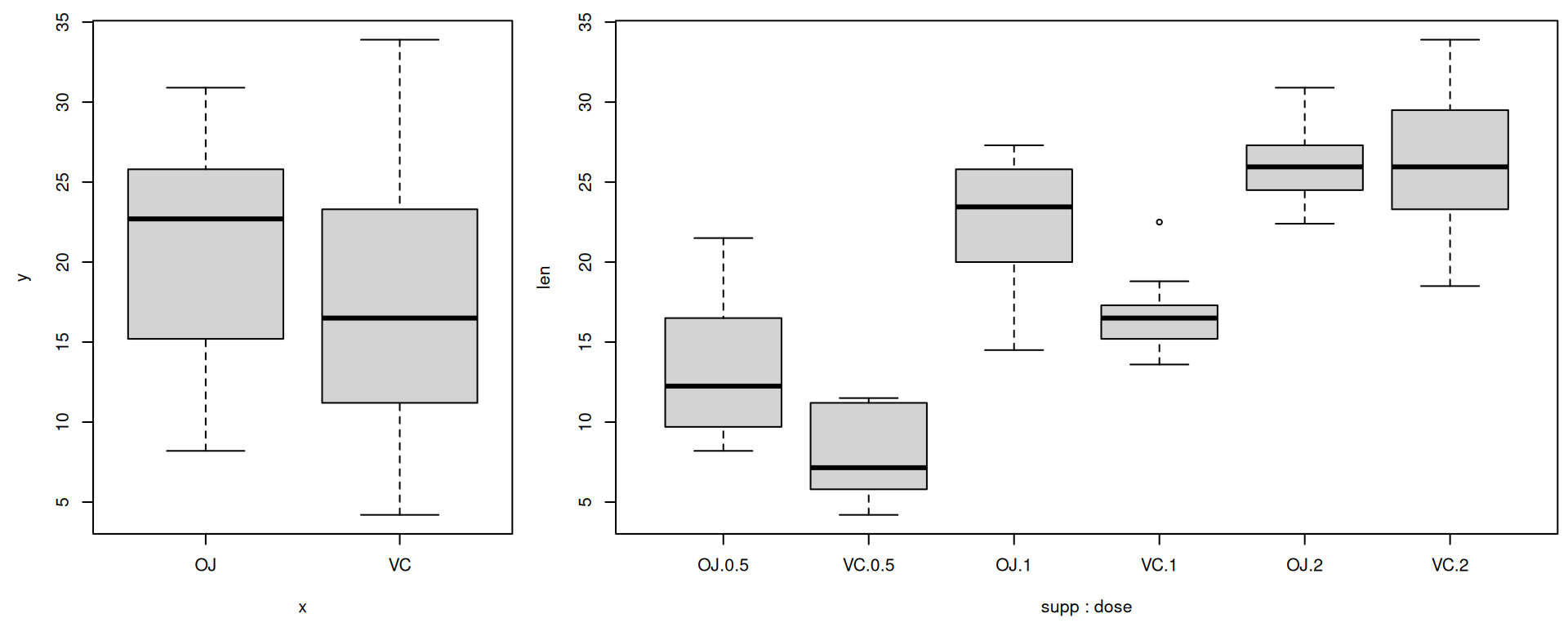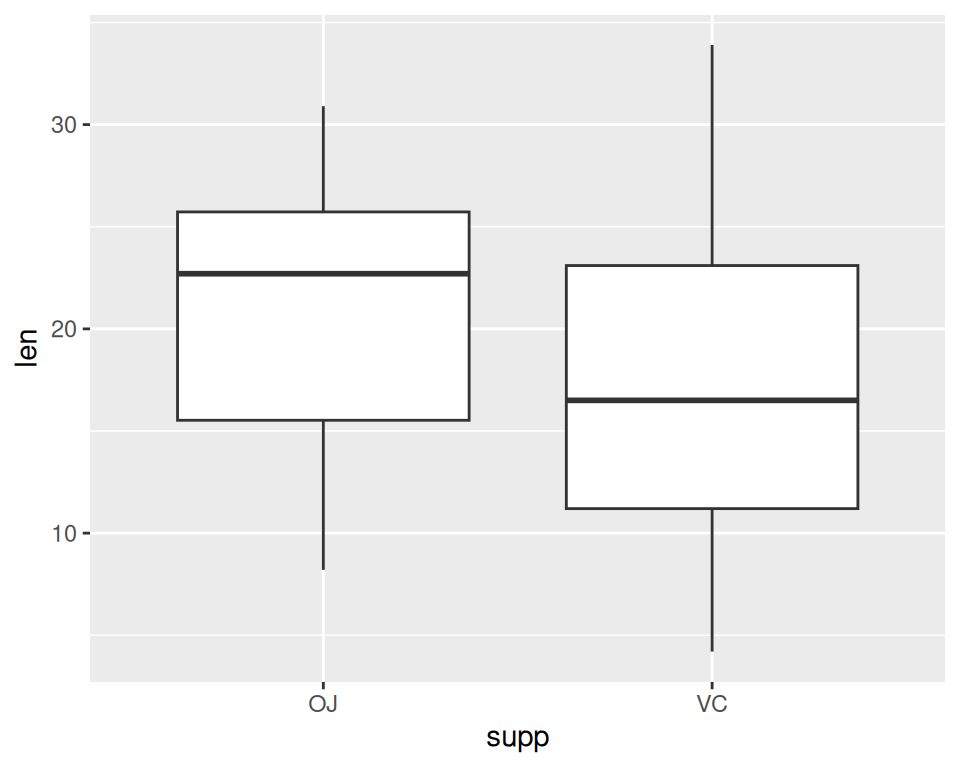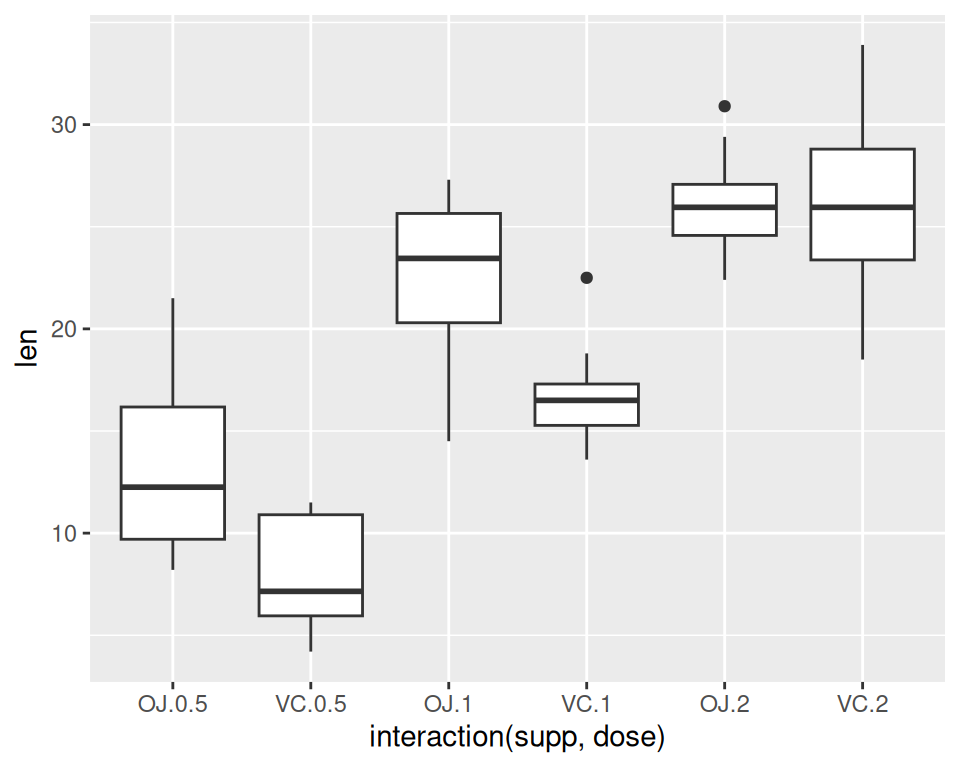## 2.5 Creating a Box Plot

### 2.5.1 Problem

You want to create a box plot for comparing distributions.

### 2.5.2 Solution

To make a box plot (Figure 2.10), use `plot()` and pass it a factor of x values and a vector of y values. When x is a factor (as opposed to a numeric vector), it will automatically create a box plot:

``plot(ToothGrowth\$supp, ToothGrowth\$len)``Figure 2.10: Box plot with base graphics (left); With multiple grouping variables (right)

If the two vectors are in the same data frame, you can also use the `boxplot()` function with formula syntax. With this syntax, you can combine two variables on the x-axis, as in Figure 2.10:

``````# Formula syntax
boxplot(len ~ supp, data = ToothGrowth)

# Put interaction of two variables on x-axis
boxplot(len ~ supp + dose, data = ToothGrowth)``````

With the ggplot2 package, you can get a similar result (Figure 2.11), with `geom_boxplot()`:

``````library(ggplot2)
ggplot(ToothGrowth, aes(x = supp, y = len)) +
geom_boxplot()``````Figure 2.11: Box plot with `ggplot()` (left); With multiple grouping variables (right)

It’s also possible to make box plots for multiple variables, by combining the variables with `interaction()`, as in Figure 2.11:

``````ggplot(ToothGrowth, aes(x = interaction(supp, dose), y = len)) +
geom_boxplot()``````

Note

You may have noticed that the box plots from base graphics are ever-so-slightly different from those from ggplot2. This is because they use slightly different methods for calculating quantiles. See `?geom_boxplot` and `?boxplot.stats` for more information on how they differ.